Learn you an Agda
Hello, Peano
16th Febuary 2011

# Definitions, Definitions

So, unlike the previous chapter, this chapter will actually involve some coding in Agda. We start with a module name.

module peano where

Most language tutorials start with the typical “Hello, World” example, but this is not really appropriate for a first example in Agda. Unlike other languages, which rely on a whole lot of primitive operations and special cases for basic constructs, Agda is very minimal - most of the “language constructs” are actually defined in libraries.

Agda doesn’t even have numbers built in, so the first thing we’re going to do is define them - specifically natural numbers. Natural numbers are positive integers, that is, the whole numbers starting with zero and going up. Mathematics uses the symbol ℕ to represent natural numbers, so we’re going to borrow that for our example (Another thing that sets Agda apart from other languages is its extensive use of unicode to make mathematical constructs more natural). To enter ℕ into emacs, type \bn. To enter the unicode arrow (→), type \->. I’m going to demonstrate this line by line, so bear with me.

  data ℕ : Set where

To begin, we type data ℕ. The data keyword means we’re defining a type - in this case, ℕ. For this example, we’re specifying that this type, ℕ, is of type Set (that’s what the colon means).

## Hold on a second, types have types?

If you recall the introduction, I mentioned that in Agda, types and values are treated the same way. This means that, seeing as values are given types, types are given types as well. Types are merely a special group of language terms, and in Agda, all terms have types.

Even Set (the type of our type ℕ) has a type: Set₁, which has a type Set₂, going on all the way up to infinity. We’ll touch more on what these Set types mean later, but for now you can think of Set as the type we give to all the data types we use in our program.

This infinite heirarchy of types provides an elegant solution to Russell’s Paradox . Seeing as for any ν∈ ℕ, Set ν contains only values “smaller” than ν, (for example, Set₁ cannot contain Set₁ or Set₂, only Set), Russell’s problematic set (which contains itself) cannot exist and is not admissable.

## Structural Induction

Okay, so, we’ve defined our type, but now we need to fill the type with values. While a type with no values does have its uses, a natural numbers type with no values is categorically wrong. So, the first natural number we’ll define is zero:

  data ℕ : Set where
zero : ℕ

Here we are simply declaring the term zero to be a member of our new type ℕ. We could continue to define more numbers this way:

    one   : ℕ
two   : ℕ
three : ℕ
four  : ℕ

But we’d quickly find our text editor full of definitions and we’d be no closer to defining all the natural numbers than when we started. So, we should instead refer to a strict mathematical definition. The notation I’m using here should be familiar to anyone who knows set theory and/or first-order logic - don’t panic if you don’t know these things, we’ll be developing models for similar things in Agda later, so you will be able to pick it up as we go along.

• Zero is a natural number (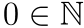).
• For any natural number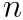,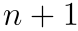is also a natural number. For convenience, We shall refer toas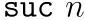1. (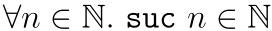).

This is called an inductive definition of natural numbers. We call it inductive because it consists of a base rule, where we define a fixed starting point, and an inductive rule that, when applied to an element of the set, induces the next element of the set. This is a very elegant way to define infinitely large sets. This way of defining natural numbers was developed by a mathematician named Giuseppe Peano, and so they’re called the Peano numbers.

We will look at inductive proof in the coming chapters, which shares a similar structure.

For the base case, we’ve already defined zero to be in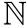by saying:

  data ℕ : Set where
zero : ℕ

For the second point (the inductive rule), it gets a little more complicated. First let’s take a look at the inductive rule definition in first order logic:This means, given a natural number n, the constructor suc will return another natural number. So, in other words, suc could be considered a function that, when given a natural number, produces the next natural number. This means that we can define the constructor suc like so:

data ℕ : Set where
zero : ℕ
suc  : ℕ → ℕ

Now we can express the number one as suc zero, and the number two as suc (suc zero), and the number three as suc (suc (suc zero)), and so on.

Incidentally, this definition of natural numbers corresponds to the Haskell data type:

data Nat = Zero | Suc Nat

If you load that into GHCi and ask it what the type of Suc is, it (unsurprisingly) will tell you: Nat -> Nat. This is a good way to get an intuition for how to define constructors in Agda.

Also, GHC supports an extension, Generalized Algebraic Data Types or GADTs, which allows you to define data types Agda style:

data Nat :: * where
Zero :: Nat
Suc  :: Nat -> Nat
It’s worth noting that GADTs are not exactly the same as Agda data definitions, and Haskell is still not dependently typed, so much of what you learn in this book won’t carry over directly to extended Haskell.

# One, Two.. Five!

Now we’re going to define some arithmetic operations on our natural numbers. Let’s try addition, first.

_+_ : ℕ → ℕ → ℕ 

Here I’m declaring a function. To start with, I give it a type2 - it takes two natural numbers, and returns a natural number.

### What do those underscores mean?

Unlike Haskell which has only prefix functions (ordinary functions) and infix functions (operators), Agda supports mixfix syntax. This allows you to declare functions where the arguments can appear anywhere within a term. You use underscores to refer to the “holes” where the arguments are meant to go.

So, an if-then-else construct in Agda can be declared with3:

if_then_else_ : ∀ { a } → Bool → a → a → a

This can be used with great flexibility: You can call this function with if a then b else c, which desugars to if_then_else_ a b c. This syntactic flexibility delivers great expressive power, but be careful about using it too much, as it can get very confusing!

Now, let’s implement this function by structural recursion4.

_+_ : ℕ → ℕ → ℕ
zero + zero = zero
zero + n    = n
(suc n) + n′ = suc (n + n′)  -- use \' to input ′.

# Our First Check

Normally we’d run the program at this point to verify that it works, but in Agda one does that pretty rarely. Instead, what we do is get Agda to check our code. This checks that all our proof obligations have been met:

• It checks your types. Types are how you encode proof obligations in Agda (although we haven’t done any non-trivial proofs yet), so this is important.
• It checks that your program provably terminates. Checking that any program terminates is in general undecidable (see The Halting Problem), but proof obligations can only be machine-checked by Agda if your program terminates. To circumvent this dilemma, Agda runs its checker only on structural recursion with finite data structures, and warns that it can’t check proof obligations if non-structural recursion is ever used. We will discuss this more in later chapters, but the only examples presented in the early part of this book will be ones that Agda can already prove terminates.

To run a check, type C-c C-l into emacs, or choose Load from the Agda menu. If your program checks correctly, there will be no error messages, no hole markers (yellow highlighting) and no orange-highlighted non-terminating sections. It should also say Agda: Checked at the bottom of the window, and you get syntax highlighting.

Right now, our checks aren’t all that meaningful - the only thing they prove is that our addition function does indeed take any two natural numbers and produce a natural number, as the type suggests. Later on, when we encode more information in our types, our checks can mean a lot more - even more than running and testing the program.

## “I Have Merely Proven It Correct”

To evaluate an expression (just to verify that it truly does work), we can type C-c C-n into emacs, or select “Evaluate term to normal form” from the Agda menu. Then, in the minibuffer, we can type an expression for 3 + 2:

(suc (suc (suc zero))) + (suc (suc zero))

And we get the result (5):

(suc (suc (suc (suc (suc zero)))))

In this chapter we have examined the Peano natural numbers, and defined some basic functions and data types in Agda. In the next chapter, we’ll look at propositional logic, and how to encode logical proofs in Agda using this system.

1. suc standing for successor.

2. Unlike Haskell, type declarations are mandatory.

3. Don’t worry if you’re scared by that ∀ sign, all will be explained in time.

4. Don’t be scared by the term - structural recursion is when a recursive function follows the structure of a recursive data type - it occurs very frequently in functional programs.